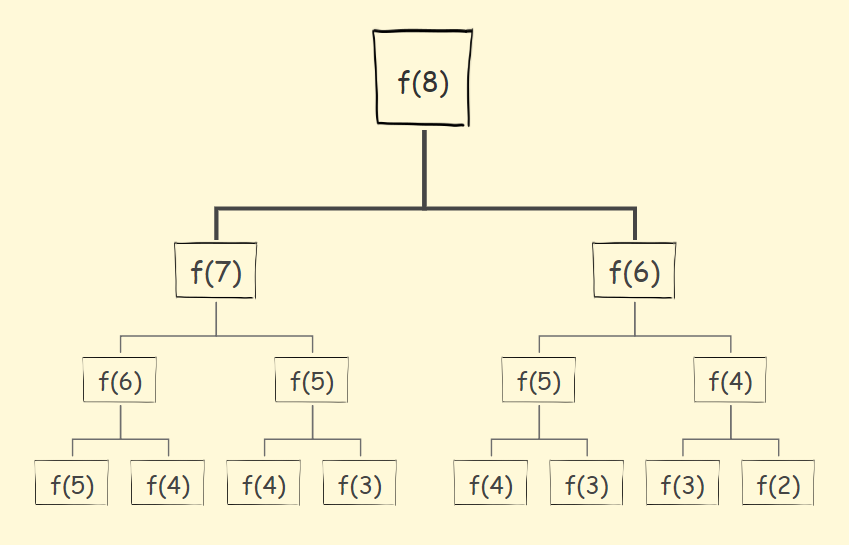# 递归

• 递归实现的原理：
一个递归函数的调用过程类似于多个函数的嵌套的调用，只不过调用函数和被调用函数是同一个函数。为了保证递归函数的正确执行，系统需设立一个工作栈。具体地说，递归调用的内部执行过程如下：
1. 运动开始时，首先为递归调用建立一个工作栈，其结构包括值参局部变量返回地址
2. 每次执行递归调用之前，把递归函数的值参局部变量的当前值以及调用后的返回地址压栈；
3. 每次递归调用结束后，将栈顶元素出栈，使相应的值参和局部变量恢复为调用前的值，然后转向返回地址指定的位置继续执行。
• 注意：在我们了解了递归的基本思想及其数学模型之后，我们如何才能写出一个漂亮的递归程序呢？我认为主要是把握好如下三个方面：
1. 明确递归函数的作用；
2. 明确递归终止条件与对应的解决办法；
3. 找出函数的等价关系式，提取重复的逻辑缩小问题规模。
• 递归三步走：
• 明确函数功能：要清楚你写这个函数是想要做什么；
• 寻找递归出口：递归一定要有结束条件，不然会永远递归下去，禁止套娃
• 找出递推关系：开始实现递归，一步一步递推出最终结果。

## 明确函数功能

``````//算n的阶乘（假设n不为0）
int f(int n) {

}``````

## 寻找递归出口（初始条件）

• 必须有一个明确的结束条件。因为递归就是有“递”“归”，所以必须又有一个明确的点，到了这个点，就不用“递下去”，而是开始“归来”。

``````//算n的阶乘（假设n不为0）
int f(int n) {
if(n == 1) {
return 1;
}
}``````

## 找出递推关系

• 初始条件：f(1) = 1
• 递推关系式：f(n) = f(n-1)*n

``````// 算n的阶乘（假设n不为0）
int f(int n) {
if(n = 1) {
return n;
}
// 把f(n)的递推关系写进去
return f(n-1) * n;
}``````

f(1)=1
f(2)=f(1)*2=2
f(3)=f(2)*3=2*3=6

# 例题

## 斐波那契数列

• 明确函数功能：f(n)为求第n项的值

``````// 1.f(n)为求第n项的值
int f(int n) {

}``````
• 寻找递归出口：f(1)=1,f(2)=1

``````// 1.f(n)为求第n项的值
int f(int n) {
// 2.递归出口
if(n <= 2) {
return 1;
}
}``````
• 找出递推关系：f(n) = f(n-1)+f(n-2)

``````// 1.f(n)为求第n项的值
int f(int n) {
// 2.递归出口
if(n <= 2) {
return 1;
}
// 3.递推关系
return f(n-1) + f(n-2);
}``````

## 小青蛙跳台阶

• 明确函数功能：f(n)为青蛙跳上一个n级的台阶总共有多少种跳法

``````int f(int n) {

}``````
• 寻找递归出口：f(0)=0,f(1)=1

``````int f(int n) {
// 递归出口
if(n <= 1) {
return n;
}
}``````
• 找出递推关系：f(n) = f(n-1)+f(n-2)

``````int f(int n) {
// 递归出口
if(n <= 2) {
return 1;
}
// 递推关系
return f(n-1) + f(n-2);
}``````

# 递归优化思路

## 自顶向下

（我比较习惯自底向上，因为比较符合数学归纳法，顺着推）## 重复计算• 优化思路：
• 建立一个数组，将子问题的计算结果保存起来。
• 判断之前是否计算过：
• 计算过，取出来用
• 没有计算过，再递归计算
• 实例：
• 把n作为数组下标，f(n)作为值。
例如arr[n] = f(n)。
• f(n)还没有计算过的时候，我们让arr[n]等于一个特殊值。
例如arr[n] = -1。
• 当我们要判断的时候，
• 如果 arr[n] = -1，则证明f(n)没有计算过；
• 否则，f(n)就已经计算过了，且f(n) = arr[n]。
直接把值取出来用就行了。

``````// 我们实现假定 arr 数组已经初始化好的了。
int f(int n) {
if(n <= 1) {
return n;
}
//先判断有没计算过
if(arr[n] != -1) {
//计算过，直接返回
return arr[n];
}else {
// 没有计算过，递归计算,并且把结果保存到 arr数组里
arr[n] = f(n-1) + f(n-1);
reutrn arr[n];
}
}``````